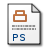# The beta-function of the Wess-Zumino model at O(1/N-2)

Ferreira, PM and
(1998) The beta-function of the Wess-Zumino model at O(1/N-2). NUCLEAR PHYSICS B, 525 (1-2). pp. 435-456.Postscript (Requires a viewer, such as GSview) 9712138v1.ps.gz - Unspecified Download (98kB)PDF 720.pdf - Unspecified Download (227kB)

## Abstract

We extend the critical point self-consistency method used to solve field theories at their d-dimensional fixed point in the large N expansion to include superfields. As an application we compute the beta-function of the Wess-Zumino model with an O(N) symmetry to O(1/N^2). This result is then used to study the effect the higher order corrections have on the radius of convergence of the 4-dimensional beta-function at this order in 1/N. The critical exponent relating to the wave function renormalization of the basic field is also computed to O(1/N^2) and is shown to be the same as that for the corresponding field in the supersymmetric O(N) sigma model in d-dimensions. We discuss how the non-renormalization theorem prevents the full critical point equivalence between both models.

Item Type: Article 19 latex pages, 5 postscript figures large N methods, critical exponents, beta-function, supersymmetry, Wess-Zumino model ?? QC ?? Faculty of Science and Engineering > School of Physical Sciences > Mathematical Sciences Symplectic Admin 02 Dec 2008 11:19 16 Dec 2022 09:50 10.1016/S0550-3213(98)00236-3 https://livrepository.liverpool.ac.uk/id/eprint/720